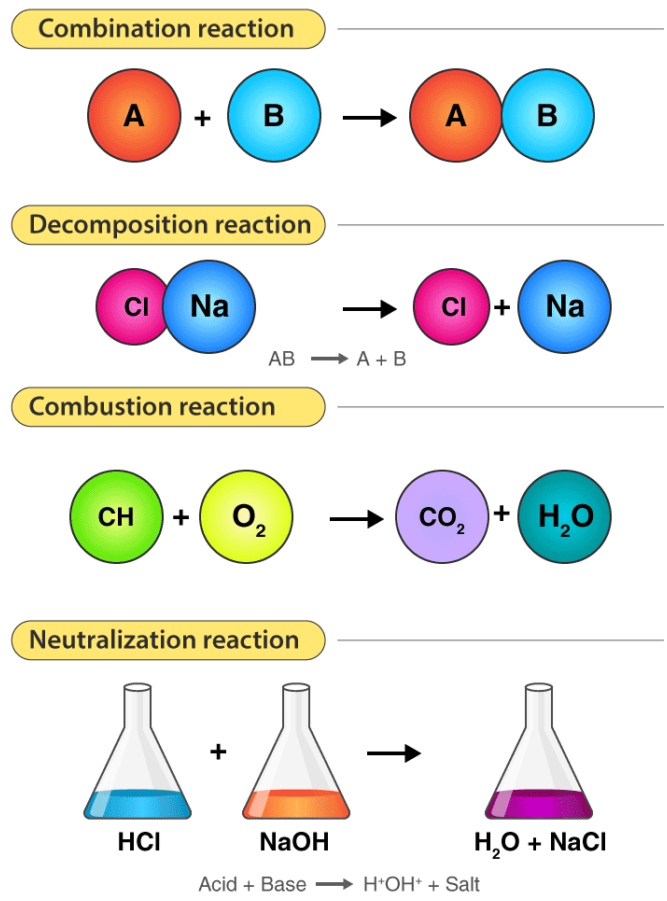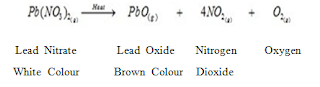# Chemical Reactions And Equations Notes – Combination, Decomposition, Displacement And Double Displacement reactionsCombination, Decomposition, Displacement And Double Displacement reactions

Types of Chemical Reactions:

i). Combination Reaction

ii). Decomposition Reaction

a). Thermal Decomposition

b). Electrolytic Decomposition

iii). Displacement Reaction OR Substitution reaction

iv). Double Displacement Reaction

a). Precipitation Reaction

b). Neutralisation Reaction

v).  Redox Reaction

vi). Oxidation Reaction

vii). Reduction Reaction

viii). Endothermic Reaction

ix). Exothermic Reaction

Explanation of the types of Chemical reactions i.e Combination, Decomposition, Displacement And Double Displacement reactions using examples:

i). Combination Reaction:

The reactions in which two or more substances combine to form a new substance are called combination reactions. For example:

a).  2 Mg (s)    +     O2 (g)     →  2 MgO

Magnesium      Oxygen         Magnesium oxide (White ash)

(basic) turns red litmus blue

b). In the laboratory, iron sulphide is prepared by mixing iron and sulphur.

Fe(s)   + S(s)    →   FeS(s)

c). Burning of carbon monoxide in oxygen to form carbon dioxide.

2CO (g)       +   O2(g)  →       2CO2 (g)

d).  Calcium oxide upon reaction with water produces calcium hydroxide.

CaO                +            H2O        →    Ca(OH)2

Calcium Oxide       Water           Calcium Hydroxide

(Quick Lime)                                (Slaked Lime)

ii). Decomposition reactions:

These are opposite to combination reactions. A single compound decomposes or break down to give two or more simpler substances.
It is of following types:

i).Thermal Decomposition:
When a decomposition reaction is carried out by heating
For Example:

• Mercuric oxide, when heated, undergoes thermal decomposition, to give mercury and oxygeniii). Displacement Reaction OR Substitution reaction

A more reactive element (metal) displaces less reactive element (metal) from its aqueous salt soln. For example:

• Zn (s)  +   FeSO4 (aq)    →    ZnSO4 (aq) + Fe (s)

(green)               (Colourless)

• Fe (s)  +  CuSO4 (aq)    →    FeSO4 (aq)  + Cu (s) ↓

Iron        Blue                   Green            Reddish Brown

• Mg (s)        +    H2SO4 (aq)     →   MgSO4 (aq) +  H2O (g)

Magnesium    Sulphuric             Magnesium     Water

Acid                        Sulphate

• Cu (s)     +     2AgNO3 (aq)      →   Cu (NO3)2 (aq)    +   2Ag (s).

Copper            Silver Nitrate      Copper Nitrate      Silver

• KI (aq)      +    Cl2 (g)           →  KCl (aq)    +    I2 (g)

Potassium     Chlorine           Potassium    Iodine

Iodide                                          Chloride

iv). Double Displacement Reaction:

The chemical reactions in which compounds react to form two different compounds by mutual exchange of ions are called double displacement reactions.

Reactions occurs by two different ways

a). Precipitation: In such reactions due to exchange of ions some insoluble material is formed. This insoluble material is called precipitate and the reaction is called precipitation reaction. For example:

• ZnSO4 (aq)    +   BaCl2 (aq)     →   ZnCl2 (aq)   +  BaSO4 (s)

Zinc                  Barium                 Zinc                Barium

Sulphate             Chloride              Chloride        Sulphate (White)

• 2 HCl (aq)      +   Pb(NO3)2(aq) →  2 HNO3 (aq)  +  PbCl2 (s)

Acid                        Nitrate                    Acid                Chloride

• Pb(NO3)2(aq)  +   2 KI (aq)        →    PbI2 (aq)   +   KNO3 (s)

Nitrate                    Iodide                 Iodide              Nitrate

b) Neutralization Reaction: In this type of reaction an acid reacts with a base to form salt and water by exchange of ions.

• NaOH (aq)   +  HCl (aq)       →      NaCl (aq)     +    H2O

Sodium          Hydrochloric       Sodium             Water

Hydroxide      Acid                        Chloride

(Base)             (Acid)                      (Salt)                (Water)

• ZnO     +     HNO3    →   Zn(NO3)2   +   H2O

Zinc            Nitric           Zinc                 Water

Oxide         Acid             Nitrate

All these types of Combination, Decomposition, Displacement and Double Displacement reactions are explained using examples along with the colour change that takes place during reactions.